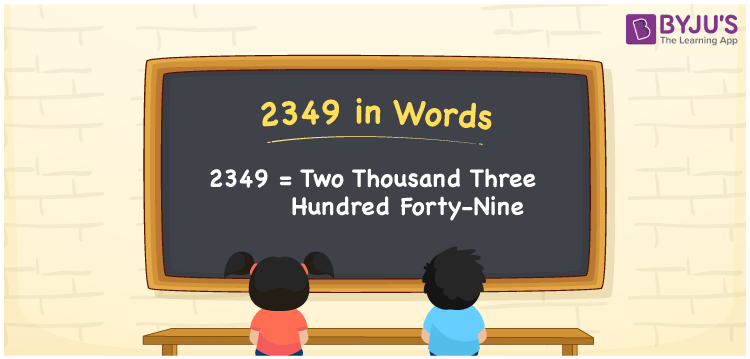# 2349 in Words

We can write 2349 in words as Two thousand three hundred forty-nine. Suppose you bought a photograph that cost Rs. 2349, you can say, “I bought a photograph for Two thousand three hundred forty-nine rupees”. It is easy to convert the given cardinal number, i.e. 2349, into words with the help of a place value chart.

 2349 in words Two thousand three hundred forty-nine Two thousand three hundred forty-nine in Numbers 2349

## 2349 in English Words

Using the English alphabet, we generally express numbers in words. Thus, we spell 2349 in words as “Two thousand three hundred forty-nine”.## How to Write 2349 in Words?

The below table depicts the place value chart for the four-digit number 2349.

 Thousands Hundreds Tens Ones 2 3 4 9

Here, ones = 9, tens = 4, hundreds = 3, and thousands = 2.

The above numbers can be expanded as:

2 × Thousand + 3 × Hundred + 4 × Ten + 9 × One

= 2 × 1000 + 3 × 100 + 4 × 10 + 9 × 1

= 2000 + 300 + 40 + 9

= Two thousand + Three hundred + Forty + Nine

= Two thousand three hundred forty-nine

Therefore, 2349 in words = Two thousand three hundred forty-nine.

Learn in detail about place value here.

As we know, 2349 is a natural number that precedes 2350 and succeeds 2348.

2349 in words – Two thousand three hundred forty-nine

Is 2349 an even number? – No

Is 2349 an odd number? – Yes

Is 2349 a prime number? – No

Is 2349 a composite number? – Yes

Is 2349 a perfect square number? – No

Is 2349 a perfect cube number? – No

## Frequently Asked Questions on 2349 in Words

Q1

### Write the number 2349 in words.

We can write the number 2349 in words as Two thousand three hundred forty-nine.
Q2

### How to write Rs. 2349 in words on a cheque?

On a cheque, we generally write Rs. 2349 in words as “Two thousand three hundred forty-nine rupees only”.
Q3

### Express the value of 2349 + 7216 in words.

2349 + 7216 = 9565 Hence, the value of 2349 + 7216, i.e., 5111 is expressed in words as Nine thousand five hundred sixty-five.